### 7 segment decoder/driver and Comparator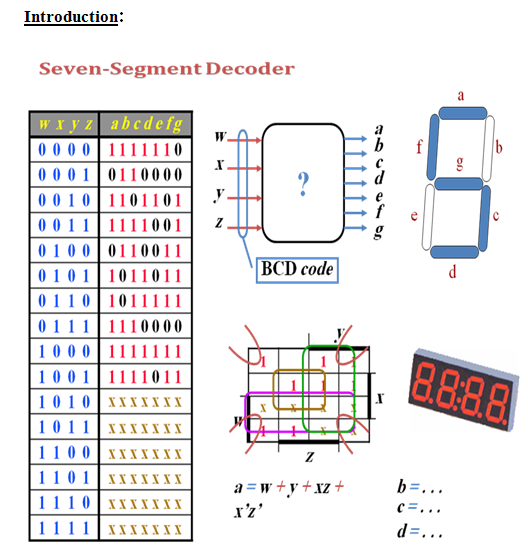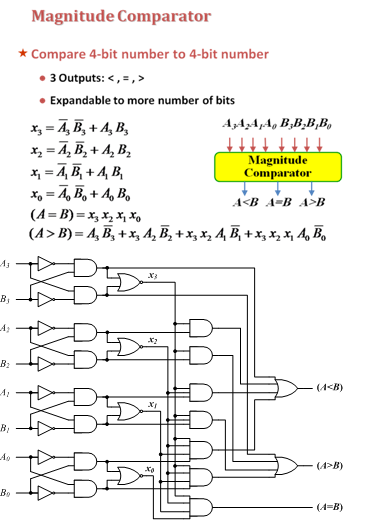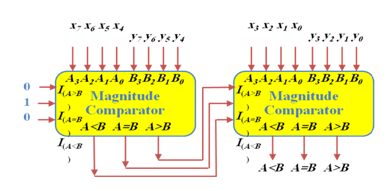Procedure:

7 segment decoder/driver:

The 7447 IC is 4 lines to 7 lines decoder used to display 7 segments displays.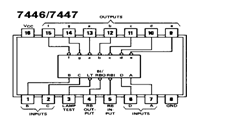Connect inputs A,B,C and D to switches and the 7 segment display to the outputs of the 7447 as shown below: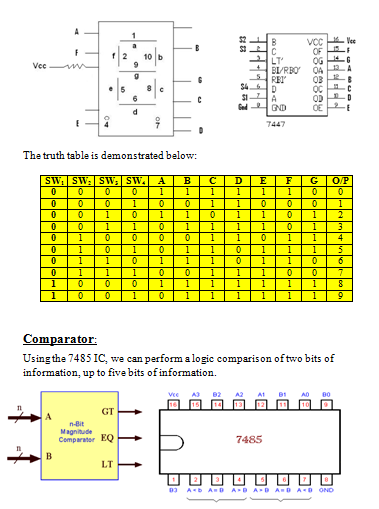We connect (A0 – A2) and (B0 - B2) inputs to switches and comparison outputs to the LEDs. Producing a 3 bit comparator as shown in the following schematic diagram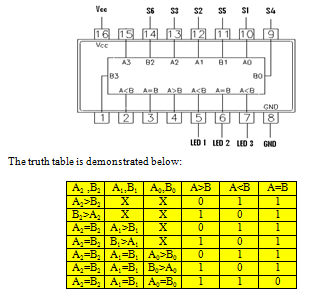Discussion:
A Magnitude comparator is a combinational logic that takes a number of binary bits as input representing two numbers  and compare their magnitude to find out if the first number =, <, or > the second number. So, if we have two 2-bits numbers that we want to compare, we need to start comparing the MSB bit first. If both MSBs are equal then we can move on to the next bits towards the LSB. For example consider comparing A = 10, and B = 11. We start by comparing A1 and B1 which are 1 and 1 in this case. It is clear that they are equal to each other. So, we can move on to the next digits to the right and compare  0 with 1 that represents A0 and B0. It is obvious that A0<B0. As a result A<B.

Conclusion:

1.     A comparator is a combinational logic that takes a number of binary bits as input representing two numbers and compare their magnitude.
2.     The seven segment display is an application of the Light Emitting Diode (LED).
3.     A strobe must be low for the device to work properly.
4.     An enable must be high for the device to work properly.